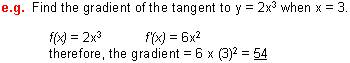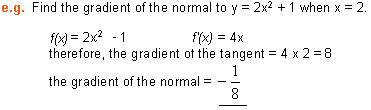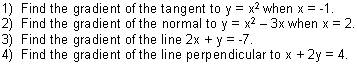# Applications of Differentiation : Math Review

math tutorials > applications of differentiation

## Derivatives & Straight Line Graphs

The derivative f'(x) is the gradient of the tangent of the graph of f at the point x.  In a straight line graph the derivative is the gradient of the graph.The normal to a curve at any point is the line perpendicular to the tangent at that point.
The gradient of two perpendicular lines has a product of –1.## Differentiation math problems for you to try.Solutions:  1)  -1    2)  -1    3)  -2    4)  2